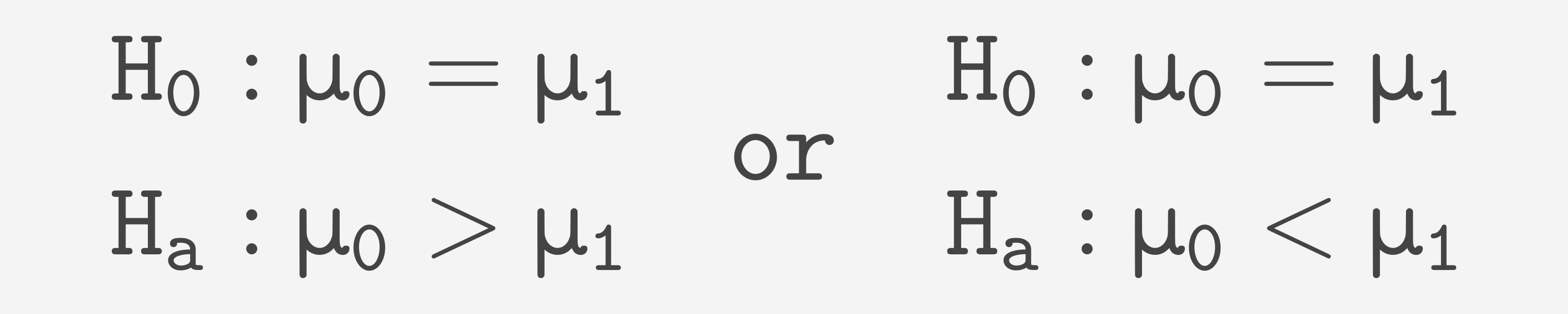Course Content

Learning Statistics with PythonHypotheses

The first step of conducting a t-test is stating the hypotheses. These are the assumptions we will confirm or disprove. Two hypotheses are required, the null hypothesis and alternative hypothesis.
The null hypothesis for a t-test is: "The means of two samples are equal."
And the alternative hypothesis is: "The means of two samples are not equal."
The null hypothesis is denoted as H₀, and the alternative hypothesis is Hₐ.If based on the t-test, we decline the null hypothesis, the alternative hypothesis is automatically accepted.

There is also another way to build an alternative hypothesis:The latter form is used when:

1. You are sure that one group has either a higher(lower) mean or the same and not the other way around.
It is the case with our heights example. We can confidently say that females are not taller than males on average.
2. You only want to know, "Is something better?" If it's not, you don't care if it is the same or worse.
For example, a new website design. You only want to deploy it if it is better than the current one, else no matter whether it is worse or the same, you will keep the current design until the new one is improved.

Section 6.

Chapter 2